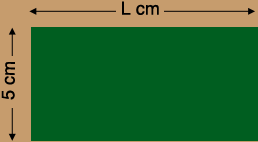SEARCH HOMEMath Central Quandaries & QueriesQuestion from Tris, a student: Hi, I'm trying to figure out the length of a rectangle but i forgot how. So, the width is 5cm and the perimeter is 34cm.Hi Tris,

Draw a diagram! In my diagram I let $L$ be the length of the rectangle.Write an expression for the perimeter of the rectangle in terms of $L.$ The value of this expression is 34 cm. Solve for $L.$

PennyMath Central is supported by the University of Regina and the Imperial Oil Foundation.